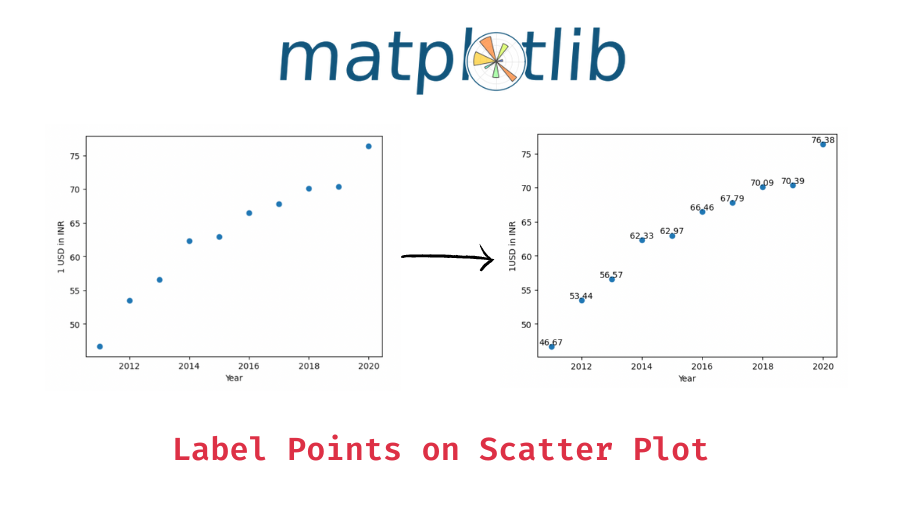# How to Label Points on a Scatter Plot in Matplotlib?

In this tutorial, we will look at how to label points on a scatter plot in Matplotlib with the help of some examples.

## How to annotate points on a scatter plot in matplotlib?

You can use the `matplotlib.pyplot.text()` function to label points in a matplotlib scatter plot. The `matplotlib.pyplot.text()` function is used to add text at the location (x, y) in the plot. The following is the syntax –

```import matplotlib.pyplot as plt

# add text, s to a point at (x, y) coordinate in a plot
plt.text(x, y, s)```

Now to add labels to each point in the scatter plot, use the `matplotlib.pyplot.text()` function for each point `(x, y)` and add its appropriate label.

Let’s now look at some examples of using the above syntax. First, we will create a simple scatter plot.

```import matplotlib.pyplot as plt

# x values - years
x = [2011, 2012, 2013, 2014, 2015, 2016, 2017, 2018, 2019, 2020]
# y values - 1 USD in INR
y = [46.67, 53.44, 56.57, 62.33, 62.97, 66.46, 67.79, 70.09, 70.39, 76.38]

# plot x and y on scatter plot
plt.scatter(x, y)

plt.xlabel('Year')
plt.ylabel('1USD in INR')```

Output:

### Example 1 – Label a point on the scatter plot

In case you only want to label a specific point (or points), use the `matplotlib.pyplot.text()` function only for those points. For example, let’s label the point `(2014, 62.33)`.

```# plot x and y on scatter plot
plt.scatter(x, y)

plt.xlabel('Year')
plt.ylabel('1USD in INR')

# add label to a point
plt.text(2014, 62.33, 'USD crosses\n60 Rupee mark')```

Output:

You can customize the way the text label appears by using additional arguments. For example, you can adjust the vertical and the horizontal alignment of the text, you can rotate the text, etc.

📚 Data Science Programs By Skill Level

Introductory

Intermediate ⭐⭐⭐

🔎 Find Data Science Programs 👨‍💻 111,889 already enrolled

Disclaimer: Data Science Parichay is reader supported. When you purchase a course through a link on this site, we may earn a small commission at no additional cost to you. Earned commissions help support this website and its team of writers.

```# plot x and y on scatter plot
plt.scatter(x, y)

plt.xlabel('Year')
plt.ylabel('1USD in INR')

# add label to a point
plt.text(2014, 62.33, 'USD crosses\n60 Rupee mark', va='center', ha='center')```

Output:

Here, we used the `va` parameter to set the vertical alignment of the text to ‘center’ and the `ha` parameter to set the horizontal alignment to ‘center’ as well.

### Example 2 – Label Each Point on the Scatter Plot

To label each point on the scatter plot, use the `matplotlib.pyplot.text()` function for each point in the plot.

```# plot x and y on scatter plot
plt.scatter(x, y)

plt.xlabel('Year')
plt.ylabel('1USD in INR')

# add labels to all points
for (xi, yi) in zip(x, y):
plt.text(xi, yi, yi, va='bottom', ha='center')```

Output:

Here, we added the y-axis value of the point as its label for each point on the scatter plot.

You might also be interested in –

•